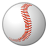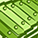# QlikView App Dev

Discussion Board for collaboration related to QlikView App Development.

Announcements
Read about the latest Qlik Community enhancements on the Community News blog!
cancel
Showing results for
Did you mean:Creator II

## Set Analysis not equal to Syntax

I am trying to use Set Analysis for a not equal to value.

=Sum({\$<[Flag]-={'x'}>}[Amount])

This is not working properly.  When I change -= to just = , I get the correct amount for the ones that should be equal to x.  How should I write this to sum the Amount where the Flag is not equal to x?

26 RepliesMaster III

May be

Sum(If(Len(Trim(Flag))=0,Amount)Not applicable

=Sum({\$<[Flag]= e({1<id={'X'}>})>}[Amount])

try thisNot applicable

corrected one

=Sum({\$<[Flag]= e({1<[Flag]={'X'}>})>}[Amount])Contributor III

Am I right ?

=Sum( {1- \$<[Flag]={'x'}> } [Amount] )Champion

Hi Cliff,

May be try,

=Sum({\$<Flag ={'*'}-{'x'}>}[Amount])Contributor II

Hi , you need to change from  " -= "    to   " =- " and it will work.

=Sum({\$<[Flag]=-{'x'}>}[Amount])Partner

Indeed, it all depends on what should be the initial selection:

• Flag -= {'x'}  Current selection minus those rows with Flag = x
• Flag =- {'x'}  All rows with flag values (including those not selected), excluding those with Flag = x.However, from the OP we cannot deduce in detail what is the initial situation...Community Browser